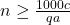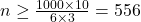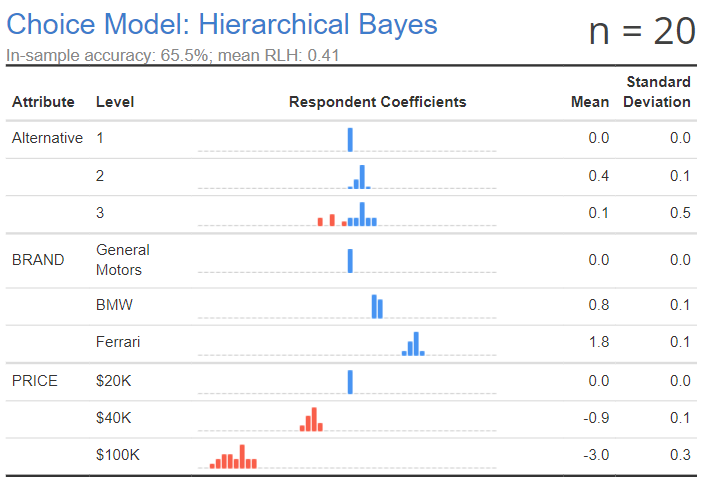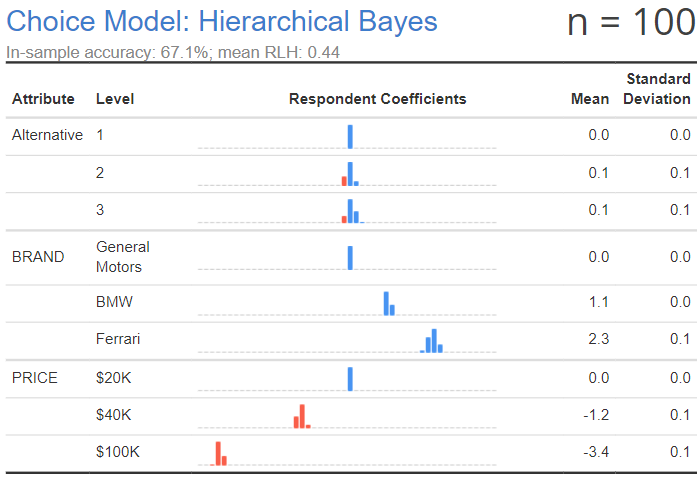# Blog27 February 2019 | by Tim Bock

# What Sample Sizes do you Need for Conjoint Analysis?

Working out the sample size required for a choice-based conjoint study is a mixture of art and science. What makes it tricky is that the required sample size depends on a number of different things:

1. The experimental design. The more alternatives in a question, the smaller the sample size that is needed. The more levels in an attribute, the bigger the sample size required; this is a big effect, but you only need to worry about the attribute with the greatest number of levels. The number of attributes can also determine the required sample size, although sometimes in counter intuitive ways (all else being equal, the more attributes, the bigger the sample size, but increasing the number of attributes can reduce the measurement error, which can make a smaller sample practical).
2. The choices that people make in the experiment. For example, if there is a “none of these” alternative and it is chosen by half the sample all the time, then you need to double your sample size. Similarly, if one attribute is particularly important to respondents, and this dominates respondents thinking when making choices, a larger sample is required in order to estimate the utilities of the other attributes’ levels. If people answer in a relatively careless way, this increases the sample size required (and may invalidate the whole experiment).
3. The underlying utilities of the attribute levels. If levels differ little in their utilities, then a larger sample is required to accurately quantify differences.
4. The research questions that the study needs to solve. If the goal of the study is just to work out which attributes are most important, a small sample size may be required. If the need is to make precise predictions about relatively unpopular brands, then a larger sample size is required.

## The minimum sample size for credibility rule

In commercial market research there are two magic numbers in widespread use for determining sample size. A sample needs to be at least 300 to be credible, and 1,000 if it is an “important” study. The choice of 300 is not quite as arbitrary as it seems. Its origin is polling research, where a sample size of 300 means that the margin of error (half the confidence interval) is less than or equal to 5.6%, which, for many real-world problems, sounds like a sufficiently small number. In a conjoint study the goal is usually to predict preference shares, which makes this rule of thumb applicable for choice modeling.

If you haven’t studied a lot of statistics, you may be perturbed by the lack of rigor in the expression “sounds like a sufficiently small number”. Surely there must be a better way than to go with whatever “sounds like a sufficiently small number?”. There is. The solution is to work out the range of possible outcomes and each of their distributions of uncertainty, compute the economic costs of each of these, and then multiply these together and compute their sum. The theory is simple. It even has a name: expected utility. In practice, however, the working out of the costs is completely impractical in most circumstances, so all that is left is to go with is “sounds like a sufficiently small number”.

## Minimum sub-group sample size

The next rule is to work out what sub-groups need to be compared, and ensure that you have a sufficient sample size in each of them. Some people are happy with sub-groups as small as 2; others choose a minimum sample size of 25 or 30, some 100, and others suggest 200 (e.g., Brian K. Orme and Keith Chrzan (2017), Becoming an Expert in Conjoint Analysis: Choice Modeling for Pros, Sawtooth Software, Inc.). For example, if there are three key sub-groups of interest, and the sample size required for each is 200, then a sample of 600 is required.

## The Sawtooth Guideline

Sawtooth Software has a guideline, adapted from some simulation work done on logistic regression (Peduzzi P, Concato J, Kemper E, Holford TR, Feinstein AR (1996) A simulation study of the number of events per variable in logistic regression analysis. Journal of Clinical Epidemiology 49:1373-1379), which says that the minimum sample size should be:where:

q is the number of questions shown to each respondent,

a is the number of alternatives per question (excluding the “none of these”),

c is the maximum number of levels of any attribute.

For example, if one attribute has 10 levels, there are 6 questions, and 3 alternatives, then:A good thing about the formula is that it shows how different decisions impact on the error of a choice model: all else being equal, it is better to have fewer levels, more questions, and more alternatives.

However, it would be a mistake to confuse the concreteness of this formula with rigor. In particular: the formula implies that if we double the number of questions asked per respondent, we can halve the sample size. Such a conclusion highlights a key conceptual problem with this formula: it mistakes sampling error (which is a function of the sample size) with measurement error (which is a function of the number of questions asked per respondent). While increasing the number of questions per respondent may reduce measurement error, its effect on the likely sampling error is hard to predict. This is most readily appreciated by thinking about the extreme case of one respondent: no matter how many questions you ask one person, the data gained from that person is still data from only one person.

## Standard errors of 0.05 or more

Another heuristic is that the standard errors for the attribute levels should be at least 0.05 when estimating a multinomial logit model. Why 0.05? It is a smallish number, and statisticians like smallish numbers that are around 0.05, presumably because it reminds them of the 0.05 level of significance. Why a multinomial logit model? Because it is a relatively simple model to use.

## Using simulations

Perhaps the most rigorous approach to determining the sample size is to:

1. Make an educated guess about the average utility of the different attribute levels. It does not matter too much if your guesses are wrong.
2. Generate simulated data consistent with your guessed average utilities.
3. Estimate a hierarchical Bayes model and do one or more of:
• Check that the model correctly estimates the means.
• Check that there is little variation about the means (unless you are simulating variation).
• Check that whatever other research conclusions are required can be accurately estimated (e.g., if the goal of the study is to estimate the preference share for a particular quantity, compute the preference share and its uncertainty).
• Check that the prediction accuracy of the model is not too high. This is more art than science, but if the prediction accuracy is over 90% there is a chance that the model is over-fitting the data.

For example, Experimental Design for Conjoint Analysis: Overview and Examples describes an experiment where the utilities of brands of car are assumed to be 0 for General Motors, 1 for BMW, and 2 for Ferrari, and the utilities of prices are 0 for $20K, -1 for$40K, and -3 for $100K. The table below shows the estimated Mean utility for a sample of 20, and we can see that it is not massively wrong. However, it is not without problems. We have estimated a utility for alternative 2, which was assumed in the experiment to have no effect (this attribute is just the position of the alternatives when shown in the questionnaire, and should have no effect). We are also estimating some variation around alternative 3 and$100K, neither of which was assumed by the experiment.At a sample size of 100, the resulting model is much better.At a sample size of 400, it doesn’t seem to have improved much at all, suggesting that 100 is as good as 400. However, 400 is still far from perfect, and it is making the same errors that occurred with the sample size of 100 (non-0 estimates for the alternatives, and all the parameter estimates being 10% to 20% larger than the assumed estimates).The previous outputs were conducted with an experimental design using 10 questions per respondent and three alternatives. The output below uses 20 questions per respondent and four alternatives. Despite only having a sample size of 100, it does a much better job at estimating the assumed utilities than the larger sample size of 400. As mentioned at the beginning of the post, the required sample size is specific to the experimental design.#### Author: Tim Bock

Tim Bock is the founder of Displayr. Tim is a data scientist, who has consulted, published academic papers, and won awards, for problems/techniques as diverse as neural networks, mixture models, data fusion, market segmentation, IPO pricing, small sample research, and data visualization. He has conducted data science projects for numerous companies, including Pfizer, Coca Cola, ACNielsen, KFC, Weight Watchers, Unilever, and Nestle. He is also the founder of Q www.qresearchsoftware.com, a data science product designed for survey research, which is used by all the world’s seven largest market research consultancies. He studied econometrics, maths, and marketing, and has a University Medal and PhD from the University of New South Wales (Australia’s leading research university), where he was an adjunct member of staff for 15 years.

Categories